PRINTABLE FOR KIDS

XII (12) HSC

XI (11) FYJC
X (10) SSC

### Practice Set 4.2 Financial Planning Class 10th Mathematics Part 1 MHB Solution

Practice Set 4.2

1. 'Chetana Store' paid total GST of Rs. 1,00,500 at the time of purchase and collected…
2. Nazama is a proprietor of a firm, registered under GST. She has paid GST of Rs. 12,500…
3. Amir Enterprise purchased chocolate sauce bottles and paid GST of Rs. 3800. He sold…
4. Malik Gas Agency (Chandigarh Union Territory) purchased some gas cylinders for…
5. M/s Beauty Products paid 18% GST on cosmetics worth Rs. 6000 and sold to a customer for…
6. Prepare Business to Consumer (B2C) tax invoice using given information. Write the name…
7. Prepare Business to Business (B2B) Tax Invoice as per the details given below. name of…

###### Practice Set 4.2
Question 1.

'Chetana Store' paid total GST of Rs. 1,00,500 at the time of purchase and collected GST Rs. 1,22,500 at the time of sale during 1st of July 2017 to 31st July 2017. Find the GST payable by Chetana Stores.

Output Tax (tax collected at the time of sale) = Rs. 1,22,500

Input Tax (tax paid at the time of purchase) = Rs. 1,00,500

∴ Input Tax Credit, ITC = Rs. 1, 00, 500

We know that GST Payable = Output Tax – ITC

⇒ GST Payable by Chetana stores = 1, 22, 500 – 1, 00, 500

= Rs. 22, 000

Question 2.

Nazama is a proprietor of a firm, registered under GST. She has paid GST of Rs. 12,500 on purchase and collected Rs. 14,750 on sale. What is the amount of ITC to be claimed? What is the amount of GST payable?

Output Tax (tax collected at the time of sale) = Rs. 14,750

Input Tax (tax paid at the time of purchase) = Rs. 12,500

∴ Input Tax Credit, ITC = Rs. 12, 500

We know that GST Payable = Output Tax – ITC

⇒ GST Payable = 14, 750 – 12, 500

= Rs. 2, 250

Question 3.

Amir Enterprise purchased chocolate sauce bottles and paid GST of Rs. 3800. He sold those bottles to Akbari Bros. and collected GST of Rs. 4100. Mayank Food Corner purchased these bottles from Akabari Bros and paid GST of Rs. 4500. Find the amount of GST payable at every stage of trading and hence find payable CGST and SGST.

Amir Enterprise:

Input GST = Rs.3800

Output GST = Rs. 4100

∴ GST Payable = Output Tax – ITC

= 4100 – 3800 = Rs. 300

We know that CGST and SGST are components of GST.

CGST is always equal to SGST

i.e. GST = CGST + SGST

Let CGST and SGST be x.

Then GST = x + x

⇒ 300 = 2x

⇒ x = 150

∴ CGST, SGST = Rs. 150

Akbari Bros:

Input GST = Rs. 4100

Output GST = Rs. 4500

∴ GST Payable = Output Tax – ITC

= 4500 – 4100 = Rs. 400

We know that CGST and SGST are components of GST.

CGST is always equal to SGST

i.e. GST = CGST + SGST

Let CGST and SGST be x.

Then GST = x + x

⇒ 400 = 2x

⇒ x = 200

∴ CGST, SGST = Rs. 200

Question 4.

Malik Gas Agency (Chandigarh Union Territory) purchased some gas cylinders for industrial use for Rs. 24,500, and sold them to the local customers for Rs. 26,500. Find the GST to be paid at the rate of 5% and hence the CGST and UTGST to be paid for this transaction. (for Union Territories there is UTGST instead of SGST.)

Input Tax = 5% of 24,500

= Rs. 1225

Output Tax = 5% of 26, 500

= Rs. 1325

We know that GST Payable = Output Tax – ITC

∴ GST Payable = 1325 – 1225

= Rs. 100

We know that CGST and SGST are components of GST.

CGST is always equal to SGST

i.e. GST = CGST + UTGST

Let CGST and UTGST be x.

Then GST = x + x

⇒ 100 = 2x

⇒ x = 50

∴ CGST, UTGST = Rs. 50

Question 5.

M/s Beauty Products paid 18% GST on cosmetics worth Rs. 6000 and sold to a customer for Rs. 10,000. What are the amounts of CGST and SGST shown in the tax invoice issued?

We know that CGST and SGST are components of GST.

CGST is always equal to SGST

i.e. GST = CGST + SGST

Let CGST and SGST be x.

Then GST = x + x

⇒ 18 = 2x

⇒ x = 9%

∴ CGST, SGST = 9%

CGST = 9% of 10, 000 = Rs. 900

∴ SGST = Rs. 900

Question 6.

Prepare Business to Consumer (B2C) tax invoice using given information. Write the name of the supplier, address, state, Date, invoice number, GSTIN etc. as per your choice. Supplier : M/s - - -- - Address- - - - - State - - - - - Date - - - - - - - Invoice No. - - - - - GSTIN - - - - - - - - - - - - - -

Particulars –

Rate of Mobile Battery - Rs. 200 Rate of GST 12% HSN 8507, 1 pc.

Rate of Headphone - Rs. 750 Rate of GST 18% HSN 8518, 1 pc.

Mobile Battery:

Taxable Value = Rs. 200

We know that CGST and SGST are components of GST.

CGST is always equal to SGST

i.e. GST = CGST + SGST

Let CGST and SGST be x.

Then GST = x + x

⇒ 12 = 2x

⇒ x = 6%

Hence CGST, SGST = 6%

We know that CGST =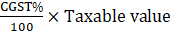⇒ CGST =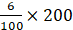= Rs. 12

∵ CGST = SGST

∴ SGST = Rs. 12

We know that Total Value = Taxable value + CGST + SGST

∴ Total Value = 200 + 12 + 12 = Rs. 224

Taxable Value = Rs. 750

We know that CGST and SGST are components of GST.

CGST is always equal to SGST

i.e. GST = CGST + SGST

Let CGST and SGST be x.

Then GST = x + x

⇒ 18 = 2x

⇒ x = 9%

Hence CGST, SGST = 9%

We know that CGST =⇒ CGST =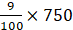= Rs. 67.5

∵ CGST = SGST

∴ SGST = Rs. 67.5

We know that Total Value = Taxable value + CGST + SGST

∴ Total Value = 750 + 67.5 + 67.5 = Rs. 885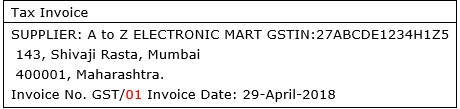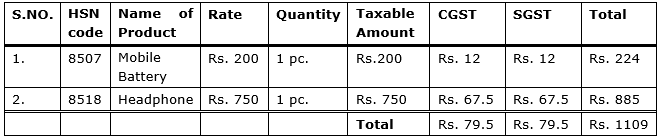Question 7.

Prepare Business to Business (B2B) Tax Invoice as per the details given below. name of the supplier, address, Date etc. as per your choice.

Supplier - Name, Address, State, GSTIN, Invoice No., Date

Recipient - Name, Address, State, GSTIN,

Items :

(1) Pencil boxes 100, HSN - 3924, Rate - Rs. 20, GST 12%

(2) Jigsaw Puzzles 50, HSN 9503, Rate - Rs. 100 GST 12%.

Pencil Box:

Taxable Value = Rs. 2000

We know that CGST and SGST are components of GST.

CGST is always equal to SGST

i.e. GST = CGST + SGST

Let CGST and SGST be x.

Then GST = x + x

⇒ 12 = 2x

⇒ x = 6%

Hence CGST, SGST = 6%

We know that CGST =⇒ CGST =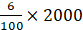= Rs. 120

∵ CGST = SGST

∴ SGST = Rs. 120

We know that Total Value = Taxable value + CGST + SGST

∴ Total Value = 2000 + 120 + 120 = Rs. 2240

Jigsaw puzzle:

Taxable Value = Rs. 5000

We know that CGST and SGST are components of GST.

CGST is always equal to SGST

i.e. GST = CGST + SGST

Let CGST and SGST be x.

Then GST = x + x

⇒ 12 = 2x

⇒ x = 6%

Hence CGST, SGST = 6%

We know that CGST =⇒ CGST =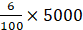= Rs. 300

∵ CGST = SGST

∴ SGST = Rs. 300

We know that Total Value = Taxable value + CGST + SGST

∴ Total Value = 5000 + 300 + 300 = Rs. 5600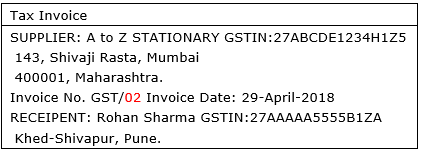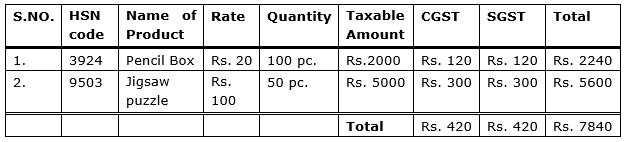## PDF FILE TO YOUR EMAIL IMMEDIATELY PURCHASE NOTES & PAPER SOLUTION. @ Rs. 50/- each (GST extra)

SUBJECTS

HINDI ENTIRE PAPER SOLUTION

MARATHI PAPER SOLUTION
SSC MATHS I PAPER SOLUTION
SSC MATHS II PAPER SOLUTION
SSC SCIENCE I PAPER SOLUTION
SSC SCIENCE II PAPER SOLUTION
SSC ENGLISH PAPER SOLUTION
SSC & HSC ENGLISH WRITING SKILL
HSC ACCOUNTS NOTES
HSC OCM NOTES
HSC ECONOMICS NOTES
HSC SECRETARIAL PRACTICE NOTES

2019 Board Paper Solution

HSC ENGLISH SET A 2019 21st February, 2019

HSC ENGLISH SET B 2019 21st February, 2019

HSC ENGLISH SET C 2019 21st February, 2019

HSC ENGLISH SET D 2019 21st February, 2019

SECRETARIAL PRACTICE (S.P) 2019 25th February, 2019

HSC XII PHYSICS 2019 25th February, 2019

CHEMISTRY XII HSC SOLUTION 27th, February, 2019

OCM PAPER SOLUTION 2019 27th, February, 2019

HSC MATHS PAPER SOLUTION COMMERCE, 2nd March, 2019

HSC MATHS PAPER SOLUTION SCIENCE 2nd, March, 2019

SSC ENGLISH STD 10 5TH MARCH, 2019.

HSC XII ACCOUNTS 2019 6th March, 2019

HSC XII BIOLOGY 2019 6TH March, 2019

HSC XII ECONOMICS 9Th March 2019

SSC Maths I March 2019 Solution 10th Standard11th, March, 2019

SSC MATHS II MARCH 2019 SOLUTION 10TH STD.13th March, 2019

SSC SCIENCE I MARCH 2019 SOLUTION 10TH STD. 15th March, 2019.

SSC SCIENCE II MARCH 2019 SOLUTION 10TH STD. 18th March, 2019.

SSC SOCIAL SCIENCE I MARCH 2019 SOLUTION20th March, 2019

SSC SOCIAL SCIENCE II MARCH 2019 SOLUTION, 22nd March, 2019

XII CBSE - BOARD - MARCH - 2019 ENGLISH - QP + SOLUTIONS, 2nd March, 2019

HSC Maharashtra Board Papers 2020

(Std 12th English Medium)

HSC ECONOMICS MARCH 2020

HSC OCM MARCH 2020

HSC ACCOUNTS MARCH 2020

HSC S.P. MARCH 2020

HSC ENGLISH MARCH 2020

HSC HINDI MARCH 2020

HSC MARATHI MARCH 2020

HSC MATHS MARCH 2020

SSC Maharashtra Board Papers 2020

(Std 10th English Medium)

English MARCH 2020

HindI MARCH 2020

Hindi (Composite) MARCH 2020

Marathi MARCH 2020

Mathematics (Paper 1) MARCH 2020

Mathematics (Paper 2) MARCH 2020

Sanskrit MARCH 2020

Important-formula

THANKS